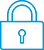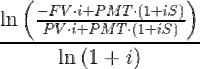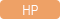cancel
Showing results for
Search instead for
Did you mean:It has been a while since anyone has replied. Simply ask a new question if you would like to start the discussion again.New member
2 1 0 0
Message 1 of 6
3,089
Flag Post

# Problems solving for N (number of periods) on HP I2cHP Recommended
HP 12c

Hello! I'm trying to solve for n with my HP12c, and I'm having a lot of trouble. It automatically rounds up to the next period (e.g. n = 3.14, the calculator returns n = 4). I've seen HP's reasoning for this design on other forums, but for my Finance classes, I need the exact answer. I've seen that there might be a way to hard code this into the calculator, but I haven't seen a thread on the HP forums yet. Any idea how to solve this problem? Thanks!

Tags (6)
5 REPLIES 5Level 6
305 297 32 76
Message 2 of 6
Flag PostHP Recommended

Perhaps the easiest solution would be to buy another calculator.

The HP12c and HP12c platinum both exhibit this feature.

The HP30b does not round n, nor HP Prime, but Prime is not a financial calculator but has a nice and user-friendly Finance app for Time Value of Money calculations.

There is a possibilty though to get the exact n.

On page 186 of the user Guide we find the formula for compound interest.

http://www.calculatrices-hp.com/uploads/pdf_en/12c_users_guide_English.pdfS is a value which can be 0 or 1.

When payments are done at ends of periods S=0, done at the beginning  S=1.

Note however that i is not exactly the same as i on the calculator.

Suppose the interest per year is 12 percent, then i on the calculator is 1, but in the formula i should be 1/100.

We want to solve this equation for n

This is rather easy. When we call (1+i)^-n=x, the equation is an easy linear equation in x, so x can be readily solved.

When we know what x is, we only have to take the LN on both sides to solve for n.

The solution is:You could store this formula into a program, replacing eg PMT by RCL PMT, because we have to read the memories.

The formula needs S, so you could store a 0 or1 into for instance memory 1, and replace S in the formula by RCL 1.Level 6
252 251 42 77
Message 3 of 6
Flag PostHP Recommended

Hello,

I am sorry, the HP 12C is an older design and does things the way things were done at the time (and also, as you probably know since you have looked at other forums where this is discussed, in a way that actually makes sense).

For some reason, your finance teacher wants the "mathematic" answer, he probably does not evern realise that said answer does not make sence in "real life"... But the 12C does not give that answer (at least not by default, there are ways, but they require more keystrokes and calculations. for example, you could first calculate the number of periods (integer), and then calcualte NFV at period -1, place than back in PV and then redo the calculations based on periods that are days long and not month long)....

If you have to have these non integer number of periods, you will need to either switch to another finance calculator (10BII+ does it as your teacher wants, other hp calcs such as the HP Prime does it too and has a good finance app), or you will need to fudge things on the 12C (you could even implement the N calculations forumla in a program).

Cyrille

I am an HP EmployeeLevel 6
305 297 32 76
Message 4 of 6
Flag PostHP Recommended

I have no financial background but my opinion is this:

I agree with Cyrille and think the way the HP12c does it is even better than giving a so called exact answer.

Let's consider the situation of a mortgage, where the final value is 0.

It is of course nonsense to pay this off in 34.459 periods, therefore this is automatically rounded to 35 periods and automatically stored in n.

When we next press FV we do net get 0 anymore but a positive value, because the calculation now is done with n=35.

This positive value is exactly the amount we payed too much in 35 periods, so the last period should be less than the periodic amount by this value.

This is very well explained  on pages 39 until 41 of the User Guide.Level 8
747 744 121 187
Message 5 of 6
Flag PostHP Recommended

Also of note is that in EVERY exam that allows the 12C, the 12C answer is acceptable and not incorrect. That may not help your situation, but the 12C is how it is "really done" in the real world. Hence why they designed it that way.

Here is the HP provided program to do your "solve for N" as a real non-fractional number. Just key that in and follow the instructions on use and you'll be able to do it just fine I suspect.

https://support.hp.com/us-en/document/bpia5043#AbT9

TW

Although I work for the HP calculator group as a head developer of the HP Prime, the views and opinions I post here are my own.New member
2 1 0 0
Message 6 of 6
Flag PostHP Recommended

Thanks for the help! I'm gonna check out that site and hopefully get a resolution. I know that once I finish this course the 12c will do everything I need.

† The opinions expressed above are the personal opinions of the authors, not of HP. By using this site, you accept the Terms of Use and Rules of Participation